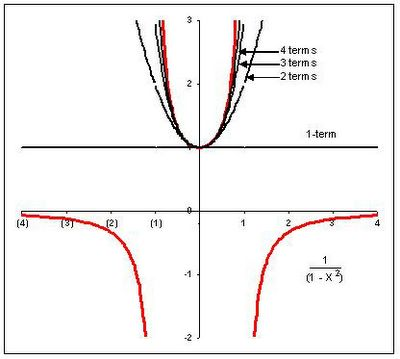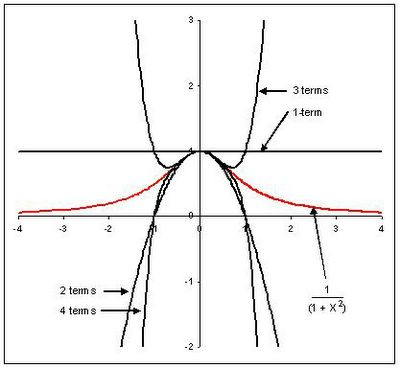## Jun 15, 2005

### part 2 - exploring attributes of functions

To really understand a mathematical function you really need to utilize imaginary numbers. I've talked before about using Fourier expansions and Taylor's Series as a way to rewrite functions so that they are easier to use or to extract certain kinds of information out of them. Let's talk about two specific examples of rewriting functions. The two functions I want to look at are
1/(1 + x2) and 1/(1 - x2)
Both of these functions can be rewritten as power series. Power series are an expansion that involve an infinite number of terms characterized by xn where n is some integer. A generic power series looks like this,
1 + Axn + Bx2n + Cx3n + Dx4n...
Both of the functions I want to consider can be rewritten as power series. Remember infinite expansions like this are typically an approximation of the original function unless you use an infinite number of terms. If you don't use an infinite number of terms then the expansion usually does a good job of representing the original equation around a specific area (e.g., around x=0). The power series look like this
1/(1 - x2) = 1 + x2 + x4 + x6...
1/(1 + x2) = 1 - x2 + x4 - x6...
Note the minus signs in the second expansion. Let's look at the first function and compare the actual function to its expansion to see how adding terms in the power series improves the accuracy of the expansion. Here's a plot of the function (in red) as well as the power series using 1, 2, 3, and 4 terms (in black).This is a decidedly unsatisfactory fit to our original function. Even though we keep adding terms the approximation only seems to work for the segment when 'x' is greater than -1 and less than 1. Why is that? Well if we look at our original function or look at the graph above it's pretty clear to see. There's a huge discontinuity in the function when x=-1 or when x=1. Plugging in those values gives us a function value of infinity (1/0). In fact there is not only a discontinuity in the actual plot, but it's clear to see that there's a discontinuity in the slope as well. The function is just too disjointed for us to adequately approximate with a power series which is by its very nature very 'continuous'. And in general this is true - functions with discontinuities like this just don't lend themselves well to approximating decompositions like Fourier or Taylor.

How about our other function - 1/(x2 + 1). If we just look at the form of the function we can see we no longer have this infinity, division by zero problem. Everything seems to be above water on this function. No discontinuities that we have to worry about. So how does it do?Well we can see again we have a problem. If we look at the plot in red of the function itself everything looks fine. It's clearly continuous and the slopes change continuously as we move along x. But the power series again breaks down near -1 and 1. The form of the power series causes us to swing wildly back and forth between the positive and negative values of y. What's going on here? You may have already guessed. If we look at this function along the imaginary axis rather than the real axis as we've done here you are again going to get a discontinuity at i and -i. You get division by zero again. It's fairly clear from this example that the function itself isn't limiting itself to the real axis. If it did we should see the power series fit well. It's not confined to the real axis. It exists on that two dimensional plane we discussed earlier. This should help you feel a little more accepting of imaginary numbers if non-imaginary functions are affected by what happens in the imaginary part of the plane.

In general when considering these discontinuities we need to look in the real/imaginary plane for them. Whenever we find a discontinuity we can draw a circle around the center of the plane (0,0) that intersects this/these discontinuity/ies. This circle is known as the circle of convergence. Inside this circle our power series will match the function well. Outside of this circle, things break down. In our second example above this circle is just a unit circle (radius 1 around the origin). That is why our power series breaks down at 1 and -1.

Next we'll talk a little about higher order mathematical operations of imaginary numbers and we'll see where e(iπ) + 1 = 0 comes from and more importantly understand how to think about it in a visual way.